# The two-formulas intro

## riPEER estimator

mdpeer provides penalized regression method riPEER() to estimate a linear model: $y = X\beta + Zb + \varepsilon$ where:

• $$y$$ - response
• $$X$$ - input data matrix
• $$Z$$ - input data matrix
• $$\beta$$ - regression coefficients, not penalized in estimation process
• $$b$$ - regression coefficients, penalized in estimation process and for whom there is, possibly1, a prior graph of similarity / graph of connections available

riPEER() estimation method uses a penalty being a linear combination of a graph-based and ridge penalty terms:

$\widehat{\beta}, \widehat{b}= \underset{\beta,b}{arg \; min}\left \{ (y - X\beta - Zb)^T(y - X\beta - Zb) + \lambda_Qb^TQb + \lambda_Rb^Tb\right \},$ where:

• $$Q$$ - a graph-originated penalty matrix; typically: a graph Laplacian matrix,
• $$\lambda_Q$$ - regularization parameter for a graph-based penalty term
• $$\lambda_R$$ - regularization parameter for ridge penalty term

## A word about the riPEER penalty properties

• A graph-originated penalty matrix $$Q$$ allows imposing similarity between coefficients of variables which are similar (or connected), based on some graph given.

• Adding ridge penalty term, $$\lambda_Rb^Tb$$, even with very small $$\lambda_R$$, eliminates computational issues arising from singularity in a graph-originated penalty matrix.

• Also, in cases when the graph information given is only partially informative / not informative about regression coefficients, ridge penalty provides partial / full regularization in the estimation.

## A cool thing about regularization parameters selection

They are estimated automatically as ML estimators of equivalent Linear Mixed Models optimization problem (see: Karas et al. (2017)).

# Examples

## example 1: riPEER used with informative graph information

library(mdpeer)

n  <- 100
p1 <- 10
p2 <- 40
p  <- p1 + p2

A <- matrix(rep(0, p*p), nrow = p, ncol = p)
A[1:p1, 1:p1] <- 1
A[(p1+1):p, (p1+1):p] <- 1
diag(A) <- rep(0,p)

# Compute graph Laplacian matrix

vizu.mat(A, "graph adjacency matrix", 9); vizu.mat(L, "graph Laplacian matrix", 9)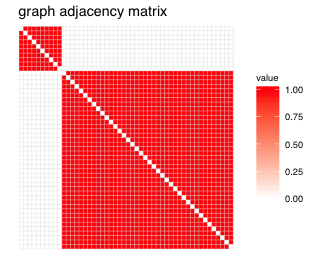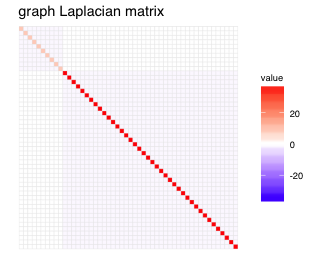• graph adjacency matrix represents connections between p=100 nodes on a graph (speaking graph-constrained regression language: represents connections / similarity between p=100 regression coefficients $$b$$)

• 1 value of $$[ij]$$ adjacency matrix entry denotes $$i$$-th and $$j$$-th nodes are connected on a graph; 0 value means they are not

• generally, adjacency matrices with continuous (both positive and negative) values might be used

# simulate data objects
set.seed(1)
Z <- scale(matrix(rnorm(n*p), nrow = n, ncol = p))
X <- cbind(1, scale(matrix(rnorm(n*3), nrow = n, ncol = 3))) # cbind with 1s col. for intercept
b.true <- c(rep(1,p1), rep(0,p2)) # coefficients of interest (penalized)
beta.true <- c(0, runif(3)) # intercept=0 and 3 coefficients (non-penalized)
eta <- Z %*% b.true + X %*% beta.true
R2 <- 0.5                         # assumed variance explained
sd.eps <- sqrt(var(eta) * (1 - R2) / R2)
error <- rnorm(n, sd = sd.eps)
y <- eta + error

$$b$$ estimation

• riPEER: graph Laplacian matrix L used as a penalty matrix $$Q$$
• riPEER: graph highly informative about regression coefficients -> relatively high contribution of graph-based penalty over contribution of ridge penalty (lambda.Q >> lambda.R)
# estimate with riPEER
riPEER.out   <- riPEER(Q = L, y = y, Z = Z, X = X, verbose = FALSE)
b.est.riPEER <- riPEER.out$b.est # (graph penalty regulatization parameter, ridge penalty regularization parameter) c(riPEER.out$lambda.Q, riPEER.out$lambda.R) ##  14.92846 1.83456 # estimate with cv.glmnet library(glmnet) cv.out.glmnet <- cv.glmnet(x = cbind(X,Z), y = y, alpha = 0, intercept = FALSE) b.est.cv.glmnet <- unlist(coef(cv.out.glmnet))[5:54] # exclude intercept and covs $$b$$ estimates plot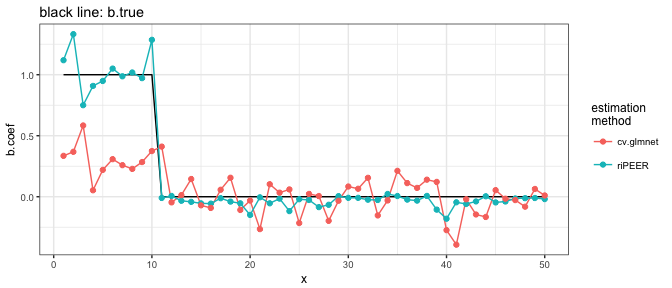$$b$$ estimation error MSEr <- function(b.true, b.est) sum((b.true-b.est)^2)/sum(b.true^2) # (riPEER error, cv.glmnet error) c(MSEr(b.true,b.est.riPEER), MSEr(b.true,b.est.cv.glmnet)) ##  0.04080697 0.59165654 ## example 2: riPEER used with non-informative graph information • use same graph information as above • simulate truen $$b$$ coefficients about which the graph given is not informative # simulate data objects set.seed(1) Z <- scale(matrix(rnorm(n*p), nrow = n, ncol = p)) X <- cbind(1, scale(matrix(rnorm(n*3), nrow = n, ncol = 3))) b.true <- rep(c(-1,1), p/2) # coefficients of interest (penalized) beta.true <- c(0, runif(3)) # intercept=0 and 3 coefficients (non-penalized) eta <- Z %*% b.true + X %*% beta.true R2 <- 0.5 # assumed variance explained sd.eps <- sqrt(var(eta) * (1 - R2) / R2) error <- rnorm(n, sd = sd.eps) y <- eta + error $$b$$ estimation • riPEER: graph non-informative about regression coefficients -> relatively high contribution of ridge penalty over contribution of graph-based penalty (lambda.Q << lambda.R) # estimate with riPEER riPEER.out <- riPEER(Q = L, y = y, Z = Z, X = X, verbose = FALSE) b.est.riPEER <- riPEER.out$b.est
c(riPEER.out$lambda.Q, riPEER.out$lambda.R)
##   1.44594 14.96809
# estimate with cv.glmnet
cv.out.glmnet <- cv.glmnet(x = cbind(X,Z), y = y, alpha = 0, intercept = FALSE)
b.est.cv.glmnet <- unlist(coef(cv.out.glmnet))[5:54] # exclude intercept and covs

$$b$$ estimates plot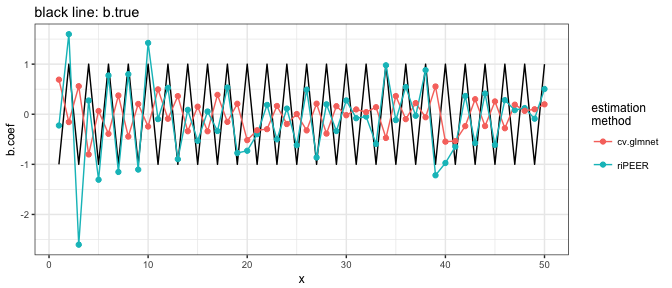$$b$$ estimation error

MSEr <- function(b.true, b.est) sum((b.true-b.est)^2)/sum(b.true^2)

# (riPEER error, cv.glmnet error)
c(MSEr(b.true,b.est.riPEER), MSEr(b.true,b.est.cv.glmnet))
##  0.5596557 1.5799423

## example 3: riPEERc used as ordinary ridge estimator

• riPEERc - method for Graph-constrained regression with addition of a small ridge term to handle the non-invertibility of a graph Laplacian matrix

• one might provide diagonal matrix as its Q argument to use it as ordinary ridge estimator

# example based on glmnet::cv.glmnet CRAN documentation
set.seed(1010)
n=1000;p=100
nzc=trunc(p/10)
x=matrix(rnorm(n*p),n,p)
beta=rnorm(nzc)
fx= x[,seq(nzc)] %*% beta
eps=rnorm(n)*5
y=drop(fx+eps)
set.seed(1011)
cvob1=cv.glmnet(x,y, alpha = 0)
est.cv.glmnet <- coef(cvob1)[-c(1)] # exclude intercept and covs

# use riPEERc (X has column of 1s to represent intercept in a model)
riPEERc.out <- riPEERc(Q = diag(p), y = matrix(y), Z = x, X = matrix(rep(1,n)))
est.riPEERc <- riPEERc.out\$b.est

$$b$$ estimates plot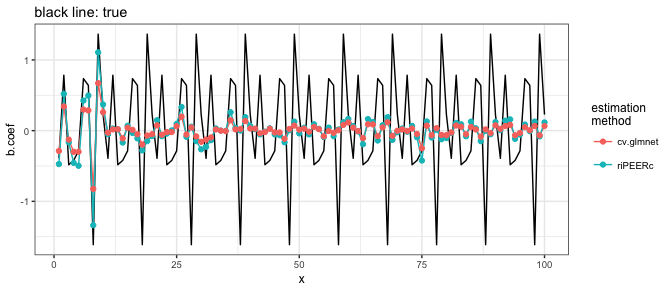$$b$$ estimation error

MSEr <- function(b.true, b.est) sum((b.true-b.est)^2)/sum(b.true^2)

# (riPEER error, cv.glmnet error)
c(MSEr(beta,est.riPEERc), MSEr(beta,est.cv.glmnet))
##  9.398393 9.415188

## Conclusion

• Example 1: graph highly informative about regression coefficients: riPEER outperforms cv.glmnet in $$b$$ coefficients estimation significantly

• Example 2: graph non-informative about regression coefficients: riPEER still outperforms cv.glmnet in $$b$$ coefficients estimation

• Example 3: riPEERc used as ordinary ridge estimator (with regularization parameter being estimated automatically) outperforms cv.glmnet in coefficients estimation

1. riPEER might be also used as ridge regression estimator by supplying a diagonal matrix as Q argument. see: Examples. Example 3.